# Going in Circles with Sketchpad

Carol A. Marinas, Ph.D.

Mathematics & Computer Science

11300 NE 3rd Court #8

Barry University

Miami, FL  33161

cmarinas@mail.barry.edu

Geometer’s Sketchpad (GSP) has been an interactive program to create meaning in geometry for a decade.  It has mainly been used at the high school and college level but should be introduced in elementary and middle school.

In particular, middle school students can move from concrete examples of shapes to more abstract representations with Sketchpad.  Through guided activities students can learn about the Sketchpad tools, observe geometric relationships, and develop conjectures.

The following guided activities about circles start with the meaning of a circle (Figure 1) and then progress to relationships between angles and arcs (Figures 3-6).

Activity #1

1.            Draw a circle in Geometer’s Sketchpad (GSP).

2.            Draw a segment from a point on the circle to the center.  Use GSP to determine its length.

3.            Draw 2 more segments between the center and 2 other points on the circle.  Use GSP to determine their length.

4.            Have a class discussion on the similarities and differences of the results.  [The individual’s segments will be of the same length while each student will have different lengths from his/her classmates.]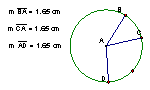Figure 1 – Radii of a Circle

Terminology: A set of points equidistant from a center point is a circle.

Terminology:  A segment joining the center with a point on the circle is the radius.  How many radii are there for each circle?  [infinitely many]

Conjecture:  Radii of the same circle are congruent.

Activity #2

1.            Draw a circle in GSP.

2.            Draw a segment with endpoints on the circle.

3.            Find the midpoint of this segment.

4.            Find the perpendicular through this midpoint.

5.            Draw a second and third segments with their endpoints on the circle.  Repeat steps 3 and 4.

6.            What do these 3 perpendicular lines have in common?  [They go through the center of a circle.]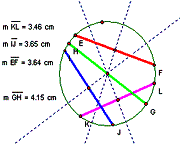# Figure 2 – Chords of a Circle

Terminology:  A segment whose endpoints are on the circle is a chord.  What is the longest chord of a circle?  [through the center of the circle]

Terminology:  A chord through the center of the circle is the diameter.  What is the relationship with the lengths of a diameter and a radius?  [The diameter is double the radius in length.]

Terminology:  A line that is perpendicular and bisects a segment is called a perpendicular bisector.

Conjecture:  Perpendicular bisectors of chords intersect at the center of the circle.

Activity #3

1.            Draw a circle and a radius. Call the center A and the other endpoint B.

2.            Create a line perpendicular line to the radius at the endpoint B.

3.            Add a point C on this perpendicular line. Measure the created angle ABC.

4.            Move the endpoint B around the circle.

5.            What conclusion can you make?  [A perpendicular line to a radius touches the circle at only one point.]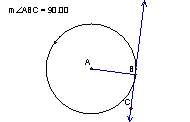# Figure 3 – Tangents to a Circle

Terminology: A line that touches a circle at only one point is called a tangent line.

Conjecture:  A tangent line is perpendicular to the radius at the point of tangency.

Activity #4

1.            Draw a circle.

2.            Create an angle that has its vertex at the circle’s center.

3.            Measure the angle.

4.            Measure the minor arc.

5.            Move the points and observe the measurements.

6.            What conclusion can you make?  [The measure of the angle whose vertex is the center of the circle equals the measure of the minor arc.]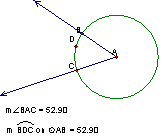# Figure 4 – Central Angles of a Circle

Terminology:  An angle whose vertex is the center of a circle is called a central angle.

Conjecture:  The measure of a central angle equals the measure of the intercepted arc.

Activity #5

1.            Draw a circle.

2.            Create an angle that has its vertex on the circle.

3.            Measure the angle.

4.            Measure the minor arc.

5.            Move the vertex around the circle.

6.            What conclusion can you make?  [The measure of the angle equals half the measure of the minor arc.]

7.            What if the angle intersected the endpoints of a diameter? [The angle is a right angle.]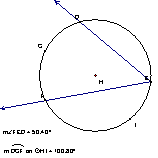# Figure 5 – Inscribed Angles of a Circle

Terminology:  An angle whose vertex is on the circle is called an inscribed angle.

Conjecture:  An inscribed angle has a measure of half the measure of the intercepted arc.

Conjecture:  An angle inscribed in a semicircle has a measure of 90 degrees.

Activity #6

1.            Draw a circle.

2.            Draw two chords that intersection in the interior of the circle.

3.            Measure the pair of acute vertical angles created at the intersection.

4.            Measure the intercepted arcs of these vertical angles.

5.            What conclusion can you make?  [The measure of the angle equals half the measure of the sum of the intercepted arcs.]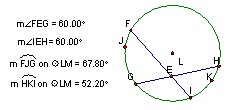# Figure 6 – Intersecting Chords in a Circle

Conjecture:  An interior angle formed by two intersecting chords has a measure that is the average of the intercepted arcs.

Activity #7

1.            Draw a circle.

2.            Draw two rays whose endpoints intersect in the exterior of the circle.

3.            Measure the angle created at the intersection.

4.            Measure the intercepted arcs of this angle.

5.            What conclusion can you make?  [The measure of the angle equals half the measure of the difference of the intercepted arcs.]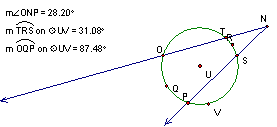Figure 7 – Rays Intersecting a Circle

Conjecture:  An angle whose vertex is in the exterior of a circle has a measure equal to half the difference of the intercepted arcs.

# Summary

Geometer’s Sketchpad can be used to:

1.                  Define geometric terms

2.                  Create conjectures about geometric relationships

3.                  Use the interactivity features of GSP to support the conjectures. (moving and animating points)

4.                  Use the table and calculate options to numerically validate the conjectures

Table Tool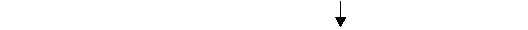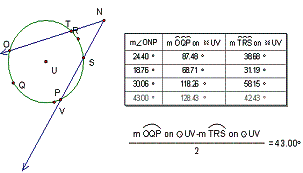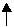### Calculator Tool

Figure 8 – Collection of Data